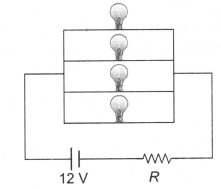Four identical electrical lamps are labelled 1.5 V 0.5 A which describes the condition necessary for them to operate at normal brightness. If a 12 V battery of negligible internal resistance is connected to lamps as shown, then

# Four identical electrical lamps are labelled 1.5 V 0.5 A which describes the condition necessary for them to operate at normal brightness. If a 12 V battery of negligible internal resistance is connected to lamps as shown, then1. A

the value of R for normal brightness of each lamp is $\left(3/4\right)\text{\hspace{0.17em}\hspace{0.17em}}\Omega$

2. B

the value of R for normal brightness of each lamp is $\left(21/4\right)\text{\hspace{0.17em}\hspace{0.17em}}\Omega$

3. C

total power dissipated in circuit when all lamps are normally bright is 24 W

4. D

power dissipated in R is 2l W when all lamps ate normally bright

Fill Out the Form for Expert Academic Guidance!l

+91

Live ClassesBooksTest SeriesSelf Learning

Verify OTP Code (required)

### Solution:

For normal brightness of each bulb see following circuit. Current through each bulb = 0.5 A

So main current  i = 2A
Also, voltage across the combination = 1.5 V
So voltage across the resistance = 10.5 V
Hence. for resistance, $\mathrm{V}=\mathrm{iR}\text{\hspace{0.17em}}⇒\text{\hspace{0.17em}\hspace{0.17em}}10.5=2\text{\hspace{0.17em}\hspace{0.17em}}×\text{\hspace{0.17em}\hspace{0.17em}}\mathrm{R}=\mathrm{R}\text{\hspace{0.17em}}=\frac{21}{4}\text{\hspace{0.17em}}\mathrm{\Omega }$

## Related content

 GK Questions on Global Warming What is Analog Computer? Types and How is it Different from Digital Computer? Possessive Nouns NEET Exam Centres in Arunachal Pradesh 2024 What is an Electric Circuit? How does it Work? GK Questions On Bihar: State Of India Plural Nouns NEET Exam Centres in Andhra Pradesh 2024 Maharashtra NEET UG Merit List 2023 5 Lesser Known Tips to Secure AIR 1 in NEET 2024+91

Live ClassesBooksTest SeriesSelf Learning

Verify OTP Code (required)